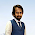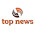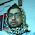### Azimuth and Elevation Angle (Antenna Look Angle) for Satellite tracking

Azimuth (Az)

The earth station needs to know where the satellite is in the orbit. Then the earth station engineer needs to calculate some angles to track the satellite correctly. These angles are called antenna look angle. The look angles for the ground station antenna are the azimuth and elevation angles required at the antenna so that it points directly at the satellite. With the geostationary orbit the situation is much simpler than any other orbit. As the antenna beam width is very narrow and tracking mechanism is required to compensate for the movement of the satellite about the nominal geostationary position. Three pieces of information that are needed to determine the look angles for the geostationary orbit are

a. Earth station latitude
b. Earth station longitude
c. Satellite orbital position

Using these information antenna look angle can be calculated using Napier’s rule (solving spherical triangle). Azimuth angle denotes the horizontal angle measured at the earth station antenna to north pole. Elevation is such angle denotes the vertical angle measured at the earth station antenna end from ground to satellite position.

Figure : Antenna azimuth angle

Equation for Azimuth (Az) determination

Here G = Difference between satellite orbital position and earth station antenna.
L = Latitude of your earth station antenna.

Elevation (El)

In the above picture Az means azimuth angle required to track the satellite horizontally. In the figure below the elevation angle has been shown.

Figure : Antenna elevation angle

Equation for Elevation (El) determination

Here 0.1512 is constant

Note
1) If the satellite orbital location is in east (E), then G = Antenna longitude  - Satellite orbital position.
2) If the satellite orbital location is in West (W), then G = Satellite orbital position - Antenna longitude

Online azimuth and elevation calculator

1.Thanks a tone ,.....its simple yet elegant

1.2.1.3.thanks to edit this one..... this side is too much
helpfull to bildup...our basse on satellites communication.....

4.This comment has been removed by the author.

1.yes brother. We always try to keep update this site. Be always with this site and keep yourself updated.

5.Some of these report features include return on investment (ROI), heaviest call times, average call length, cost per call, here unique call tracking, and it even records the conversations for quality control or training purposes.

6.While SPOT Gen 3 has the ability to send just OK o gadget trackers
r Help pre-set messages to site or fixed contact list, with Inreach there is no impediment to the informing capacity, or who messages can be sent to.

7.The customer gets the same user experience as calling 1-800-YourBusinessName and you gain the ability to measure the results of your advertising campaign. Track China Post to Canada packages tracking number